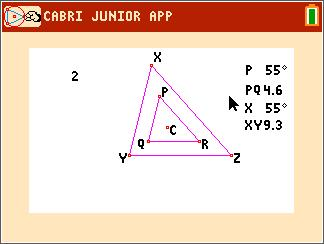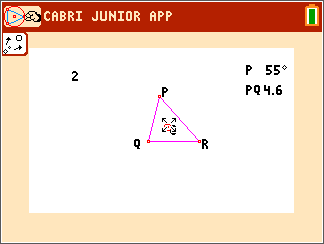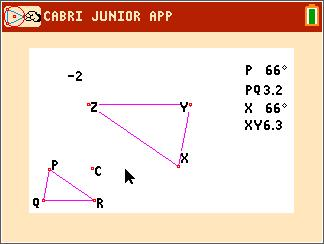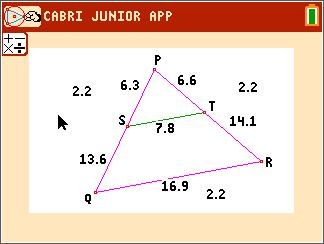# Activities

•• ##### Author45 Minutes

• ##### Report an Issue

Constructing Similar Triangles#### Activity Overview

Students investigate three different methods of constructing similar triangles.

#### Key Steps

•Students use the Cabri™ Jr. application to construct two similar triangles using the Dilation tool. By moving a point in the triangle and moving the point of dilation, students will determine whether the relationships of the angles and side measurements are preserved.

•In Problem 2, students will continue to investigate similar triangles by changing the scale factor. They will summarize their findings, stating the effects of a dilation on corresponding angles and sides.

•For the third problem, students will construct two similar triangles by constructing a line segment parallel to one of the sides of the original triangle. By showing that all three pairs of angles are congruent and all three ratios of corresponding side lengths are the same, they will prove similarity.